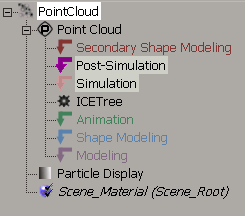An object's construction stack is divided into different regions so that operators can be evaluated in different ways that are appropriate to the task that you're doing. Traditionally, there are regions for Modeling, Animation, Shape Modeling, and Secondary Shape Modeling. If you're working with simulated ICE trees, however, there are two more construction regions that are created: Simulation and Post Simulation.The Simulation and Post Simulation regions are used only for ICE simulations and appear automatically when you create a Simulated ICE Tree node (operator). Currently, only particles and deformation operators can have simulated ICETree nodes.

For more information on the Simulation and Post-Simulation regions, see ICE Trees and the Construction Stack.

Simulated means that the result of the current frame depends on the result of the previous frame rather than on the results of the construction regions that are below it. This is what differentiates a simulation from other types of modifications to an object. For example, if you use a Push deformation on a sphere, at each frame the Push operator gets the undeformed sphere and applies the push. If you move one frame forward, the Push gets the undeformed geometry again and applies the same push.

The only way to modify the push deformation over time is to animate its parameters. However, if the Push operator is in the Simulation region, at each frame it takes the results of the sphere's pushed points from the previous frame and pushes them out more. As you play the simulated push, you see the sphere gradually inflating, without any animation being defined. The only way to return to the undeformed sphere is to go back to the first frame.

When a simulation is active, the regions below the Simulation region are not re-evaluated. This means that if you have any operators in the Animation region, those operators are not evaluated again after the simulation is active. If you want to return to using the animation operators instead of the simulation, simply select and delete the Simulation marker from the stack so that there's no Simulation region.

The Simulate Particles and Simulate Rigid Bodies Nodes

In the world of ICE, there are two nodes that allow you to simulate particles: the Simulate Particles node and the Simulate Rigid Bodies node. These are their stories.

Simulate Particles Node

The Simulate Particles node is the "standard" particles node that updates the position and velocity of each particle at each frame by doing the following. It:

• Calculates the point accelerations based on mass and force.

• Updates the point velocities based on acceleration.

• Updates the point positions based on velocity.

• Sets the simulated frame fraction to 1. The SimulatedFrameFraction attribute defines the fraction of a frame that has already been simulated for a point. Setting this value to 1 prevents points from being resimulated by other Simulate nodes in the same tree (such as the Simulate Rigid Bodies node) or in other trees on the same object.

The Emitter compounds that use this node automatically set the SimulatedFrameFraction to (1 – Age/SimulationStep) so that on its first frame of existence, a particle moves only for a distance proportional to its age. As a result, it appears as if particles have been emitted continuously throughout the frame instead of all at once.

For information on using the Simulate Particles node for emissions, see Creating a Basic Particle Emission.

The Simulate Rigid Bodies Node

The Simulate Rigid Bodies node is similar to Simulate Particles in that it simulates the particles, but it also lets you make particles into PhysX rigid bodies, which are objects that do not deform in a collision. The node updates a particle's position, velocity, orientation, and angular velocity from the previous frame based on its rigid body attributes (such as elasticity, friction, shape, size, and scale).

Using this node, particles can collide with each other, based on their shape, and with other objects that are set as obstacles. You can also create springs and dampers between points on objects that have the Simulate Rigid Bodies node in their ICE tree.

Unlike the Simulate Particles node, the Simulate Rigid Bodies node does not have the SimulateFrameFraction attribute because the PhysX dynamics engine doesn't work on frame fractions to detect collisions: it uses whole frames only.

You need to have only one Simulate node plugged into an ICETree node at a time. This means that if the Simulate Rigid Bodies node is plugged into the ICETree node, you don't need the Simulate Particles node to also be plugged in.

The only time you need the Simulate Particles node to be plugged in addition to the Simulate Rigid Bodies node is if some of the particles are acting as rigid bodies while others are acting as "regular" particles, and you switch between each type of simulation at some point (such as by using a state system or an If node and setting a condition for switching).

For information on using the Simulate Rigid Bodies node, see ICE Rigid Bodies.